ISEE Lower Level Quantitative : Tables

Example Questions

Example Question #1 : Tables

Use the following table to determine the cost of purchasing two books.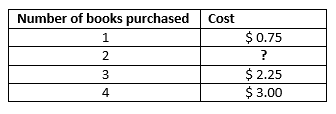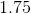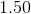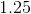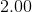Explanation:

The relationship between the values is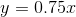,

whererepresents the cost of purchased books andrepresents the number of books purchased.

Once we realize this, we can determine how much purchasing two books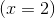would cost: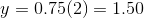Example Question #2 : Tables

Use the table to determine how much one cupcake would cost.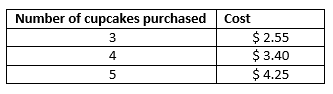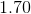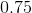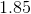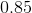Explanation:

We can determine the relationship between the values by creating a ratio of number of cupcakes to cost: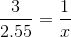Whererepresents the cost of 1 cupcake.

We can now solve for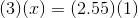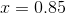The cost of one cupcake is then \$0.85

Example Question #3 : Tables

The following table consists of the test grades fromstudents. Use the table to determine how many students received aon the test.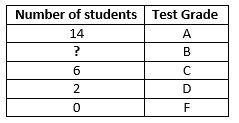Explanation:

We were told the grades are fromstudents. The most direct way to solve this problem is to add the numbers of students listed so far: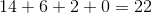We know there is a total ofstudents so we can set up the equation: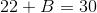which leaves us with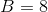So the missing value is eight.

Example Question #4 : Tables

Use the table to determine how much one cupcake would cost.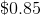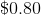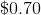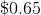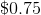Explanation:

We can determine the relationship between the values by creating a ratio of number of cupcakes to cost:Whererepresents the cost of 1 cupcake.

We can now solve forThe cost of one cupcake is then